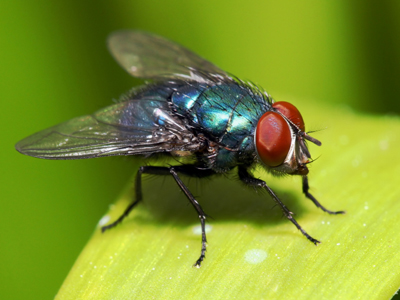You would use millimeters to measure a fly.

# Measures 2

This Math quiz is called 'Measures 2' and it has been written by teachers to help you if you are studying the subject at middle school. Playing educational quizzes is a fabulous way to learn if you are in the 6th, 7th or 8th grade - aged 11 to 14.

It costs only \$12.50 per month to play this quiz and over 3,500 others that help you with your school work. You can subscribe on the page at Join Us

As children progress through middle school they will learn more about measures in Math. As well as understanding the different values of the metric measures (for example that 2 liters = 2,000 milliliters), children will be exposed to some of the old imperial measures such as pints. They will also learn how to write measurements using more than one type of measure, for example 2.5 meters as 2m 50cm.

To measure liquid capacity, milliliters, centiliters and liters are used. These are called metric measures but we some times use other measures like gallons or pints. These are known as imperial measures. There are more imperial measures that we sometimes use - miles for long distances, stones for weights and inches for short distances instead of the metric kilometers, kilograms and centimeters.

Do you know your liters from your pints? Test your knowledge of measures by playing this quiz.

1.
How many grams are equivalent to one quarter kg?
25g
250g
250.5g
2,500g
There are 1,000g in a kg so divide by 4
2.
What is one tenth of a liter in milliliters?
0.1ml
1ml
10ml
100ml
There are 1,000 ml in a liter so divide by 10
3.
What is 6.45m expressed in meters and cm?
6m 45cm
64m 5cm
0m 645cm
645m 0cm
Here the numbers to the left of the decimal point are meters. The first two numbers to the right of the decimal point are cm. If there was a third number to the right of the decimal point then all three would be mm
4.
Which unit would you use to measure the length of a fly?
Kilometer
Millimeter
Centimeter
Meter
Flies are very small so mm would be best
5.
What is half of 1 meter in cm?
0.5cm
5cm
50cm
500cm
1m = 100cm so a half of 100 is 50
6.
Which of the following could be the weight of a small puppy, for instance a Chihuahua?
100g
10kg
100kg
50g
A larger puppy, such as a Great Dane, can weigh as much as 1kg at birth!
7.
How many millimeters in one meter?
10mm
100mm
1,000mm
10,000mm
The prefix 'milli' means the same as 11,000
8.
10 millimeters is equal to?
1cm
10cm
100cm
1,000cm
1m = 100cm = 1,000mm
9.
Which of these statements is true?
Pint > 1 liter
Pint = 1 liter
Pint > 0.5 liter
Pint = 2 liters
A pint is slightly more than half a liter
10.
How do we write 451cm in m?
0.451m
4.51m
45.1m
451m
Divide by 100 to convert centimeters to meters
Author:  Amanda Swift